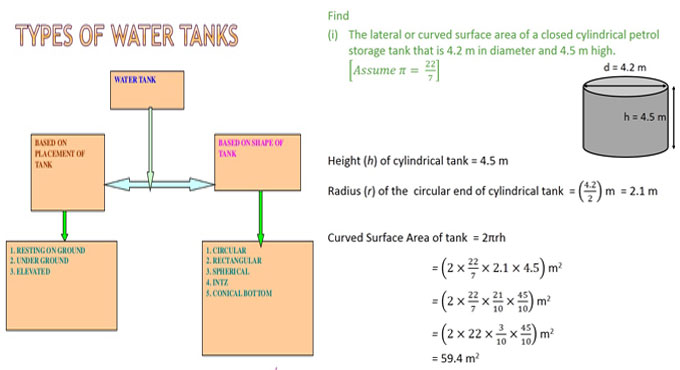# Types of water tank & the height calculation of tankOn the basis of material types and location, the water tank is categorized as follow :-

Chemical contact tank ? This type of water tank is formed with polyethylene construction and prepared for retention time of chemical treatment.

Ground water tank ? It is formed with lined carbon steel. The water is delivered through a water well or from surface water. A huge quantity of water is arranged in inventory and utilized throughout high demand cycles.

Elevated Water Tank ? This type of water tank is also called water tower. It will produce pressure at the ground level outlet containing 1 psi per 2.31 feet of elevation. Thus, a tank that is raised to 70 feet develops about 30 psi of discharge pressure. 30 psi is ideal for most domestic and industrial requirements.

Vertical cylindrical dome top ? This type of tank may reserve fifty gallons to various million gallons. These types of tanks are suitable for transport as their low-profile produces a low center of gravity facilitating to sustain equilibrium for the transport vehicle, trailer or truck.

A hydro-pneumatic tank ? This type of tank generally refers to a horizontal pressurized storage tank. By pressurizing this reservoir of water, a surge free delivery of accumulated water can be provided to the distribution system.

### On the basis of the shapes, the water tank is categorized as follow :-

Circular Tanks ? They are most cost-effective and utilized in greater capacity for delivering water, sewerage treatment etc.

Rectangular Tanks ? These types of tanks are utilized for small storage capacity and their construction process is expensive.

Spherical Tanks ? These types of tanks are utilized for the cost-cutting measure and aesthetic view point.

Intze Tanks ? They are suitable for greater storage capacity. In these types of tanks, domes are utilized as a substitute for level slabs.

### How to find out the maximum daily demand necessary for the population of the locality?

Target Population = 1500
Maximum daily consumption
= 180% of average daily demand = 1.8 x q
The demand of water for domestic purpose = 200 lit / capita / day
Average daily requirement (q) = Per demand x Population
= 200 x 1500 = 3,00,000 lit / day
So, maximum daily demand = 1.8 x q litres / day
= 1.8 x 3,00,000 litres / day
= 540 x 103 litres / day
= 540 m3 / day

### How to find out the complete height of the tank?

Suppose, the diameter of tank (D) = 12m (<30m)
Therefore, radius of tank (R) = 12/2 = 6m
Now, the volume of tank = 540 m3
The height of the cylindrical tank is taken as h
So, the capacity of tank = p x R2 x h

### After deducting the values, we get the following :-

p x 62 x h = 540
or, h = 540 / (p x 36)
or, h = 4.77
So, the height of the cylindrical tank = 4.77 m
Suppose, free boarding = 0.25 m
Therefore, the total height of the tank = (4.77 + 0.25) = 5.02 m = 5 m (approx)